# tensorly.decomposition.parafac2

parafac2(tensor_slices, rank, n_iter_max=100, init='random', svd='numpy_svd', normalize_factors=False, tol=1e-08, random_state=None, verbose=False, return_errors=False, n_iter_parafac=5)[source]

PARAFAC2 decomposition  of a third order tensor via alternating least squares (ALS)

Computes a rank-rank PARAFAC2 decomposition of the third-order tensor defined by tensor_slices. The decomposition is on the form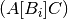such that the

i-th frontal slice,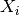, of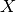is given by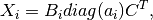where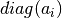is the diagonal matrix whose nonzero entries are equal to the-th row of the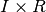factor matrix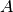,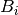is a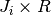factor matrix such that the cross product matrix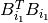is constant for all, and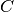is a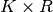factor matrix. To compute this decomposition, we reformulate the expression forsuch that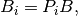where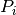is aorthogonal matrix and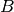is a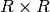matrix.

An alternative formulation of the PARAFAC2 decomposition is that the tensor element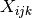is given by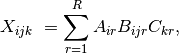with the same constraints hold foras above.

Parameters: tensor_slicesndarray or list of ndarraysEither a third order tensor or a list of second order tensors that may have different number of rows. Note that the second mode factor matrices are allowed to change over the first mode, not the third mode as some other implementations use (see note below). rankintNumber of components. n_iter_maxintMaximum number of iteration init{‘svd’, ‘random’, CPTensor, Parafac2Tensor}Type of factor matrix initialization. See initialize_factors. svdstr, default is ‘numpy_svd’function to use to compute the SVD, acceptable values in tensorly.SVD_FUNS normalize_factorsbool (optional)If True, aggregate the weights of each factor in a 1D-tensor of shape (rank, ), which will contain the norms of the factors. Note that there may be some inaccuracies in the component weights. tolfloat, optional(Default: 1e-8) Relative reconstruction error tolerance. The algorithm is considered to have found the global minimum when the reconstruction error is less than tol. random_state{None, int, np.random.RandomState} verboseint, optionalLevel of verbosity return_errorsbool, optionalActivate return of iteration errors n_iter_parafac: int, optionalNumber of PARAFAC iterations to perform for each PARAFAC2 iteration Parafac2Tensor(weight, factors, projection_matrices) weights1D array of shape (rank, )all ones if normalize_factors is False (default), weights of the (normalized) factors otherwise factorsList of factors of the CP decomposition element i is of shape(tensor.shape[i], rank) projection_matricesList of projection matrices used to create evolvingfactors. errorslistA list of reconstruction errors at each iteration of the algorithms.

Notes

This formulation of the PARAFAC2 decomposition is slightly different from the one in . The difference lies in that here, the second mode changes over the first mode, whereas in , the second mode changes over the third mode. We made this change since that means that the function accept both lists of matrices and a single nd-array as input without any reordering of the modes.

References

  (1, 2, 3) Kiers, H.A.L., ten Berge, J.M.F. and Bro, R. (1999), PARAFAC2—Part I. A direct fitting algorithm for the PARAFAC2 model. J. Chemometrics, 13: 275-294.